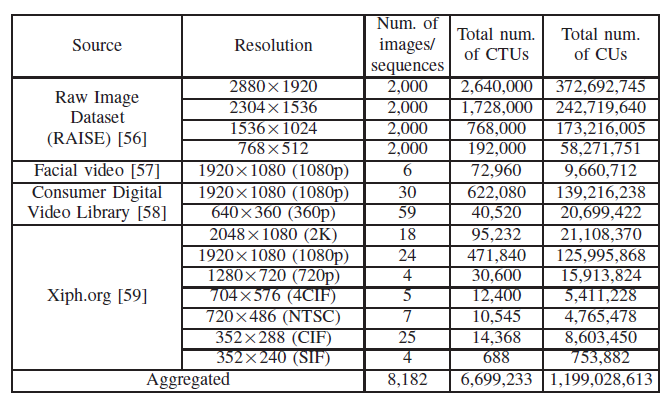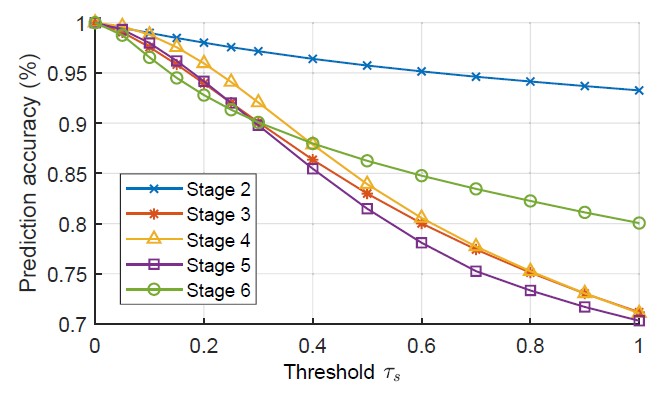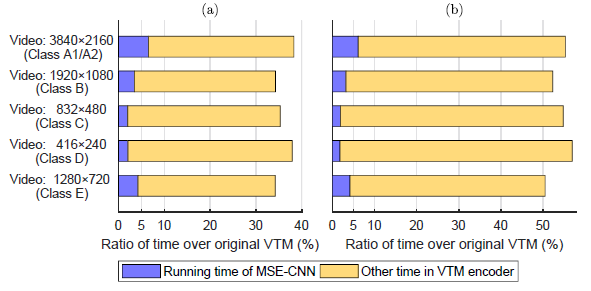# Reading: DeepQTMT — A Deep Learning Approach for Fast QTMT-based CU Partition of Intra-mode VVC (Fast VVC)

## Outperforms ETH-CNN, Reduce the Encoding Time of VVC by 44.65% — 66.88% With the Negligible BD-Rate Increase of 1.322% — 3.188%

In this story, A Deep Learning Approach for Fast QTMT-based CU Partition of Intra-mode VVC (DeepQTMT), by Beihang University, is presented. I read this because I work on video coding research. In this paper:

• First, a large-scale database containing sufficient CU partition patterns with diverse video content is established, which can facilitate the data-driven VVC complexity reduction.
• Next, a multi-stage exit CNN (MSECNN) model with an early exit mechanism is proposed to determine the CU partition, in accord with the flexible QTMT structure at multiple stages.
• Then, an adaptive loss function is designed for training the MSE-CNN model, synthesizing both the uncertain number of split modes and the target on minimized RD cost.
• Finally, a multi-threshold decision scheme is developed.

This is an article in 2020 arXiv (v1) which only appeared few days ago. (23rd June 2020). According to the article format, it should be submitted to IEEE transaction and being under review. (Sik-Ho Tsang @ Medium)

# Outline

1. CU Partition of Intra-mode VVC (CPIV) Database
2. MSE-CNN: Network Architecture
3. Loss Function
4. Multi-threshold Decision
5. Experimental Results

# 1. CU Partition of Intra-mode VVC (CPIV) Database

• The data were collected from 204 raw video sequences – and 8,000 raw images  with multiple resolutions and diverse content.
• These video sequences and images were divided into three non-overlapping sets for training (6,400 images and 160 sequences), validation (800 images and 22 sequences) and test (800 images and 22 sequences).
• VTM-7.0 is used to encode the above contents to get the samples.
• The output labels are one of six possible split modes, i.e., non-splitting (mode 0), quad-tree (mode 1), horizontal binary-tree (mode 2), vertical binary-tree (mode 3), horizontal ternary-tree (mode 4) and vertical ternary-tree (mode 5).CU Partition of Intra-mode VVC (CPIV) Database
• There are 6,699,233 samples with more than 1 million CUs, as shown above.

As shown above, ternary-tree split CUs, i.e., modes 4 and 5, account for less than 15% for all CU sizes, while non-splitting CUs, i.e., mode 0, are predominant for most CU sizes. Thus, the multi-stage CU partition problem is more sophisticated than a typical image classification problem with only one output and balanced classes.

# 2. MSE-CNN: Network Architecture

• The luminance channel of a 128×128 CTU is as input to MSE-CNN.
• In each split mode decision unit, the input feature maps first flow through a series of convolutional layers, named as conditional convolution, to extract textural features in the backbone of MSE-CNN. Then, the feature maps are fed into a sub-network to predict the split mode of one CU.
• If the prediction result is non-split, the CU partition is early-terminated at the current stage.
• Otherwise, the part of feature maps, corresponding to the location of each split CU, is input to the next stage.

## 1.1. Conditional convolution

• Instead of a fixed structure, the structure is selected on condition of the CU size.
• If the minimal axis length of current CU and that of its parent CU are ac and ap, respectively, the input feature maps are processed with nr ∈ {0, 1, 2} residual units.
• That is, different number of residual units are processed depending on the CU sizes.
• After that, the output feature maps goes through the sub-network.
• For all residual units with the same index k (though they may be at different stages), the trainable parameters are shared.

## 1.2. Sub-network

• The input feature maps flow into a series of convolutional and fully connected layers, for predicting the split mode.
• The configuration of each sub-network is related to its corresponding CU size as shown above.
• In each sub-network, the input feature maps are fed into two or three convolutional layers.
• For all convolutional layers, the width and height of their kernels are integer powers of 2, e.g., 2×2 and 4×4. And they are non-overlapping convolutions.
• Then, the output feature maps of convolutional layers flow through two fully connected layers to obtain the split mode.
• The output one-hot vector ranges from 2 to 6.
• Before the first convolutional and the first fully connected layer, QP is supplemented as an external feature.
• A half-mask operation is applied to these features, i.e., multiplying half of feature maps/vectors by the normalized QP value.
• If the CU is predicted as non-split, the partition process early exits at the current stage; otherwise, the output of conditional convolution at the current stage is fed into the next stage.
• Finally, 19 MSE-CNN models are trained according to CU sizes and color channels.

# 3. Loss Function

## 3.1. Cross Entropy Loss

• Basic cross entropy is:
• where yn,m and ˆyn,m represent the ground-truth binary label and predicted probability for the n-th CU at split mode m.
• However, the classes are unbalanced, penalty weight is applied to the basic cross entropy loss:
• where pm is the quantitative proportion of CUs with split mode m. Summing all pm for all m equals to 1.
• Additionally, α [0, 1] is an adjustable scalar to determine the importance of penalty weights.
• α = 0 means no penalty. Model may be ill trained.
• α = 1. The prior distribution is hardly learned.
• α = 0.3 is used based on the CPIV validation set.

## 3.2. RD Loss

• where rn,m is the RD cost for the n-th CU at split mode m, and rn,min is the minimum RD cost for this CU among all possible split modes.
• The ratio (rn,m/ rn,min-1) is the normalized RD cost.
• The whole term inside the summation punishes more on either larger wrongly predicted probability or larger RD cost.

## 3.3. Total Loss

• where β is a positive scalar determining the importance of the RD cost.
• β=1.

# 4. Multi-threshold Decision

• For all candidate modes m of this CU, only the modes with probability ˆyn,m ≥ τ·ˆyn,max are checked in the RDO process of the encoder.
• Different CU sizes, different values of threholds are used.Prediction accuracy of MSE-CNN on the validation data
• Different thresholds, different prediction accuracies are obtained.
• Finally, two cases are concluded:
• Based on the cases, 5 settings are selected as above.

# 5. Experimental Results

## 5.1. BD-Rate (BD-BR) & Time Difference (ΔT)

• Setting (ii) averagely reduces 59.57%-66.88% of encoding time on the video sequences, more effective than the time reduction of 55.65%-59.14% in 2019 ICME , 52.48%-64.44% in 2019 TCSVT  and 38.19%-41.79% in ETH-CNN .
• For RD performance, Setting (iv) of our approach achieves the least BD-rate redundancy of 1.322% and BD-PSNR degradation of 0.055dB on average, better than all state-of-the-art approaches ETH-CNN , 2019 ICME , 2019 TCSVT .
• Setting (ii) outperforming 2019 ICME , 2019 TCSVT  and Setting (iv) outperforming ETH-CNN  in terms of all three metrics ΔT, BD-rate and BD-PSNR.
• Lefter, Bottomer, Better.

## 5.2. Running Time AnalysisRunning time of the proposed MSE-CNN model and the VTM encoder. (a) Multi-threshold setting of (ii). (b) Multi-threshold setting of (iv).
• The time overhead introduced by MSE-CNN is less than 5% for most resolutions, compared over the original VTM. The average time overhead is 3.67%, which accounts for only a small part of the total encoding time.
• It is because of the early-exit mechanism.

This is the 38th story in this month!

--

--